Function Repository Resource:

# AntiSimplify

Make a number or expression more complicated—the opposite of Simplify

Contributed by: Wolfram|Alpha Math Team
 ResourceFunction["AntiSimplify"][expr] complicates the value of expr by essentially adding zeroes and multiplying by ones. ResourceFunction["AntiSimplify"][expr,param] complicates expr using the numeric or symbolic parameter param.

## Details

Random functions are added and multiplied to the expression to obfuscate its original value. Randomness is seeded on the expression itself, so while choices are random for each given function, repeated evaluations of ResourceFunction["AntiSimplify"] will return the same value.
ResourceFunction["AntiSimplify"] accepts the option "Complexity" which takes a positive integer value and defaults to 2. This value increases the number of times the expression is iteratively obfuscated.

## Examples

### Basic Examples (2)

Complicate a number:

 In:=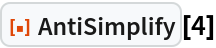Out=Complicate a symbolic expression:

 In:=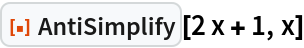Out=### Scope (2)

Use a particular value to expound upon a number:

 In:=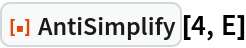Out=Simplifying the expression gives back the initial value:

 In:=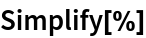Out=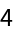Obfuscate a symbolic expression by introducing a new variable:

 In:=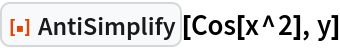Out=Again, simplifying gives back the initial value:

 In:=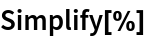Out=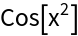### Options (4)

The "Complexity" option ramps up the number of nested obfuscation loops the function applies:

 In:=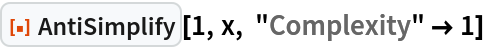Out=In:=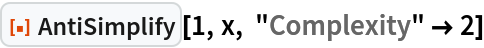Out=Even more:

 In:=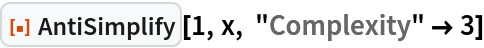Out=This can get very complicated with larger values:

 In:=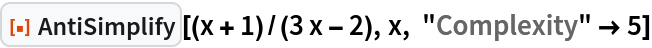Out=## Publisher

Wolfram|Alpha Math Team

## Version History

• 2.0.0 – 23 March 2023
• 1.0.0 – 28 September 2021

## Author Notes

To view the full source code for AntiSimplify, evaluate the following:

 In:=